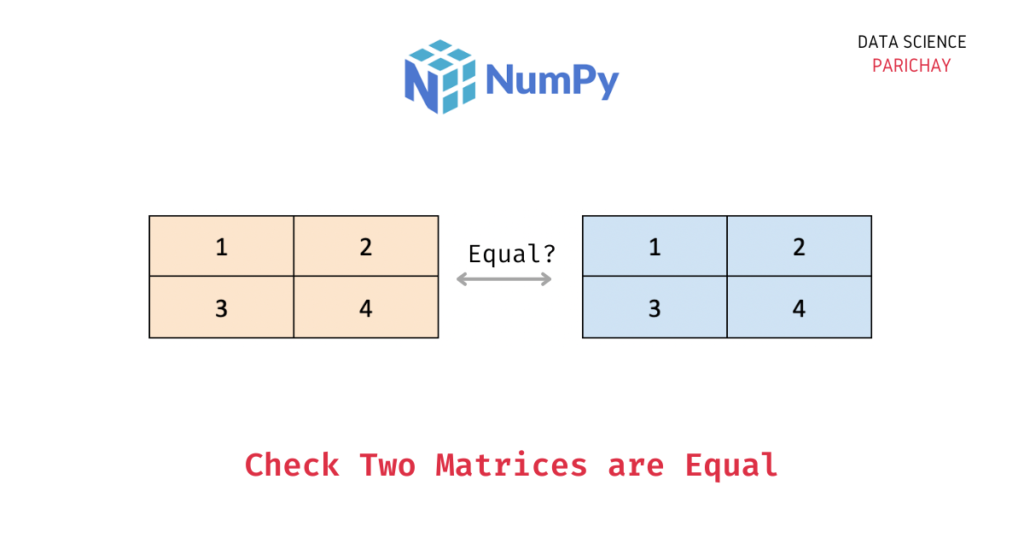# Numpy – Check If Two Matrices are Equal

In this tutorial, we will look at how to check if two matrices (2d numpy arrays) are equal or not with the help of some examples.

## How to check if two matrices are equal in numpy?

There are multiple ways using which you can assess whether two numpy arrays (including 2d arrays which we generally refer to as matrices) are equal or not.

1. Use the `numpy.array_equal()` method.
2. Compare the two matrices using the `==` operator and check if all the values in the resulting array are `True` or not.
3. Use the `numpy.allclose()` method.

Let’s now look at the above methods in detail with the help of some examples.

### Example 1 – Using the `numpy.array_equal()` method

The `numpy.array_equal()` method is used to check if two arrays are equal or not. It returns `True` if the corresponding elements in the array are exactly equal.

Let’s look at an example.

```import numpy as np

# create numpy arrays
ar1 = np.array([
[1, 2, 3],
[4, 5, 6]
])

ar2 = np.array([
[1, 2, 3],
[4, 5, 6]
])

# check if the above arrays are equal
print(np.array_equal(ar1, ar2))```

Output:

`True`

Here, we created two arrays with the same elements (in the same positions) and then checked if they are equal or not using the `numpy.array_equal()` method which returned `True`.

Let’s look at another example.

📚 Data Science Programs By Skill Level

Introductory

Intermediate ⭐⭐⭐

🔎 Find Data Science Programs 👨‍💻 111,889 already enrolled

Disclaimer: Data Science Parichay is reader supported. When you purchase a course through a link on this site, we may earn a small commission at no additional cost to you. Earned commissions help support this website and its team of writers.

```import numpy as np

# create numpy arrays
ar1 = np.array([
[1, 2, 3],
[4, 5, 6]
])

ar2 = np.array([
[1, 2, 3],
[0, 5, 6]
])

# check if the above arrays are equal
print(np.array_equal(ar1, ar2))```

Output:

`False`

We get `False` as the output because here the two matrices are not equal.

### Example 2 – Using the `==` operator

You can also use the `==` operator to check whether two matrices are equal or not. This will return a new array with the same shape as the input arrays, where each element is `True` if the corresponding elements in the input arrays are equal, and `False` otherwise.

Now, if all the values in the resulting array are `True` we can say that both the matrices are equal. Use the `numpy.all()` function to check if all the array values are `True` or not.

Let’s look at some examples.

```import numpy as np

# create numpy arrays
ar1 = np.array([
[1, 2, 3],
[4, 5, 6]
])

ar2 = np.array([
[1, 2, 3],
[4, 5, 6]
])

# check if the above arrays are equal
print(np.all(ar1 == ar2))```

Output:

`True`

We get the correct result.

Let’s look at another example, where the values are not equal.

```import numpy as np

# create numpy arrays
ar1 = np.array([
[1, 2, 3],
[4, 5, 6]
])

ar2 = np.array([
[1, 2, 3],
[0, 5, 6]
])

# check if the above arrays are equal
print(np.all(ar1 == ar2))```

Output:

`False`

We get `False` as the output.

### Example 3 – Using the `numpy.allclose()` method

Sometimes you may want to compare two arrays with values that are very close but not exactly equal and this is more common than you think. For example, when performing matrix transformations, you may get matrices with values that have rounding errors (depending on how floats are represented on your machine). For example, a value that should ideally be 1 comes out to be 0.9999999.

In such cases, you can use the `numpy.allclose()` function which returns `True` if the two input arrays are element-wise equal within a tolerance. This can be useful if the arrays contain floating point numbers, which can have rounding errors.

By default, the tolerance is set to 1e-05, which means that two values are considered equal if they are within 0.00001 of each other. You can adjust this tolerance by passing a different value to the `rtol` (relative tolerance) or `atol` (absolute tolerance) parameters of `numpy.allclose()`.

Let’s look at an example.

```import numpy as np

# create numpy arrays
ar1 = np.array([
[1, 0],
[0, 1]
])

ar2 = np.array([
[1.00001, 0],
[0, 0.99999]
])

# check if the above arrays are equal
print(np.allclose(ar1, ar2))```

Output:

`True`

We get `True` as the output for two matrices that have very close values.

You might also be interested in –

•